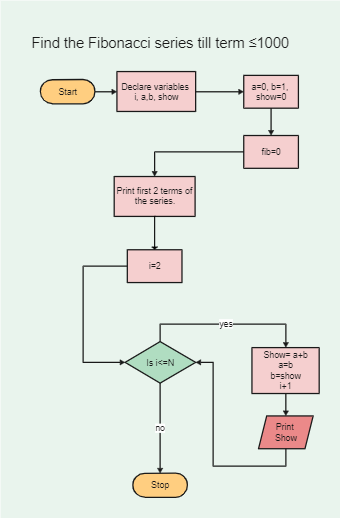Template Community / Fibonacci series Algorithm Flowchart

# Fibonacci series Algorithm FlowchartAshley
Published on 2021-09-27
Edit OnlineStep 1: Declare the variables i, a, b, show. Step 2: Enter the values for the variables, a=0, b=1, show=0 Step 3: Enter the terms of the Fibonacci series to be printed, i.e=, 1000. Step 4: Print the first two terms of the series. Step 5: Loop the following steps: -Show = a + b -a= b -b = show -Add 1 to the value of i each time. -Print Show
Tag
algorithm flowchart
Report
1
799PostRecommended Templates